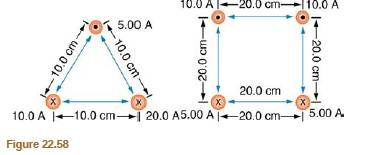Chapter 22, Problem 70PE

Chapter
Section
Textbook Problem

Find the magnitude and direction of the magnetic field at the point equidistant from the wires in Figure 22.58(b), using the rules of vector addition to sum the contributions from each wire.To determine

The magnitude and the direction of the magnetic field at the point equidistant from the wires.

Explanation

Given info:

Current through wire A is,IA=10.0A.

Current through wire B is,IB=10.0A.

Current through wire C is,IC=5.0A.

Current through wire C is,ID=5.0A.

Formula used:

General formula to calculate the magnetic field produced by a long straight wire is given as,

B=μ0I2πr       ...... (1)

Calculation:

Consider the given figure.

The equidistant point will be the center of the square formed by four wires that is pointO.

The distance of pointOfrom each wire is,

r=120.202+0.202r=14.14×102m

Substituting the values from the figure for wire A in equation (1), we get

BA=(4π×107Tm/A)(10.0A)2π(14.14×102m)BA=1.414×105T

Substituting the values from the figure for wire B in equation (1), we get

BB=(4π×107Tm/A)(10.0A)2π(14.14×102m)BB=1.414×105T

Substituting the values from the figure for wire C in equation (1), we get

BC=(4π×107Tm/A)(5

Still sussing out bartleby?

Check out a sample textbook solution.

See a sample solution

The Solution to Your Study Problems

Bartleby provides explanations to thousands of textbook problems written by our experts, many with advanced degrees!

Get Started

Find more solutions based on key concepts